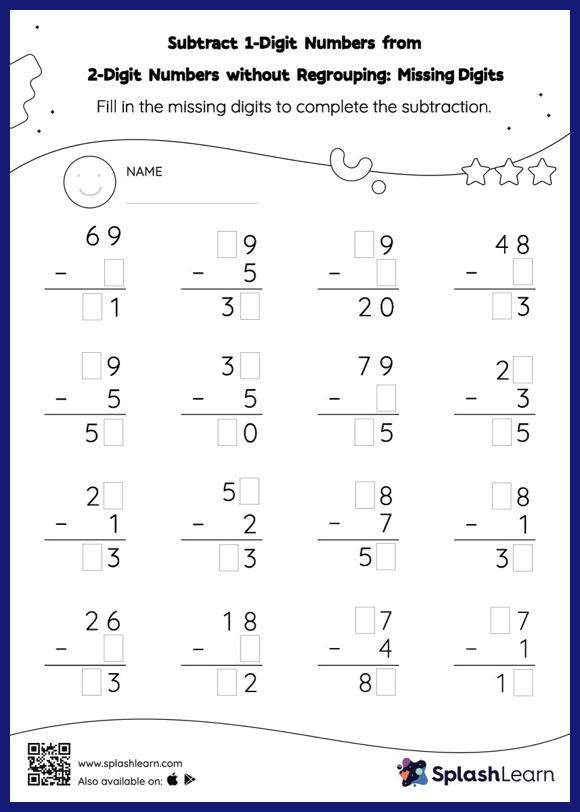# Subtract 1-Digit Numbers from 2-Digit Numbers without Regrouping: Missing Digits Worksheet

Home > Subtract 1-Digit Numbers from 2-Digit Numbers without Regrouping: Missing DigitsThis worksheet invites students to subtract 1-digit numbers from 2-digit numbers without regrouping, which helps them become proficient in subtraction. To find the missing number on this worksheet, students can utilize the count back strategy or the connection between addition and subtraction. In subtract 1-digit numbers from 2-digit numbers without regrouping worksheet, they do not need to regroup numbers to reach the solution.